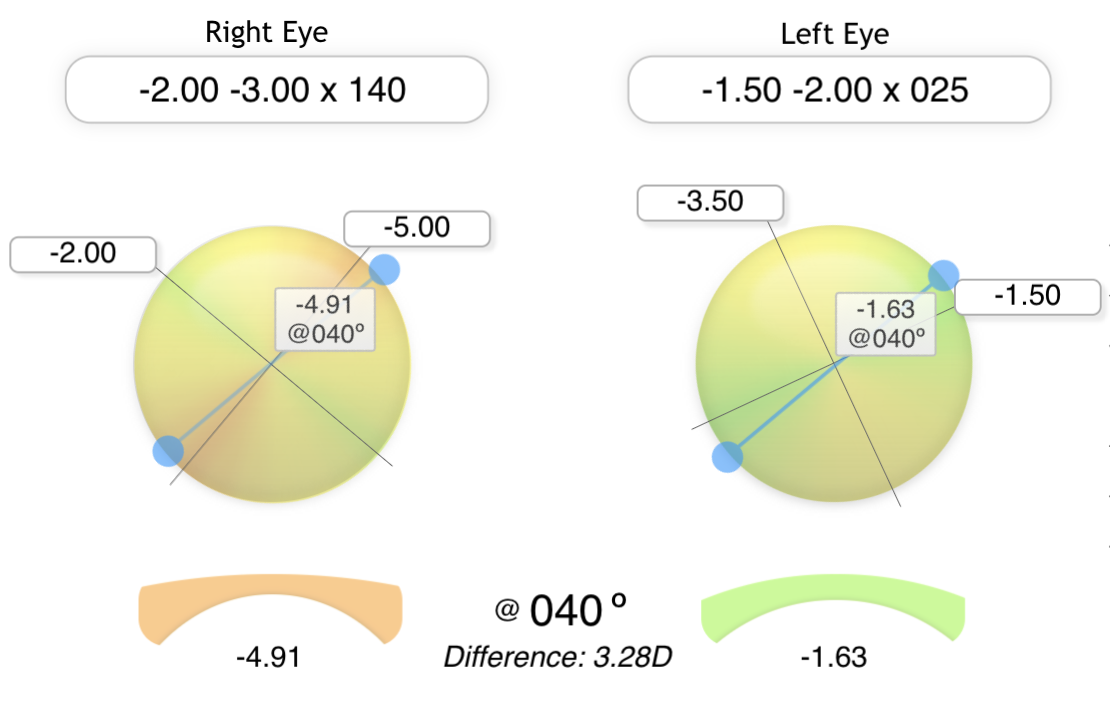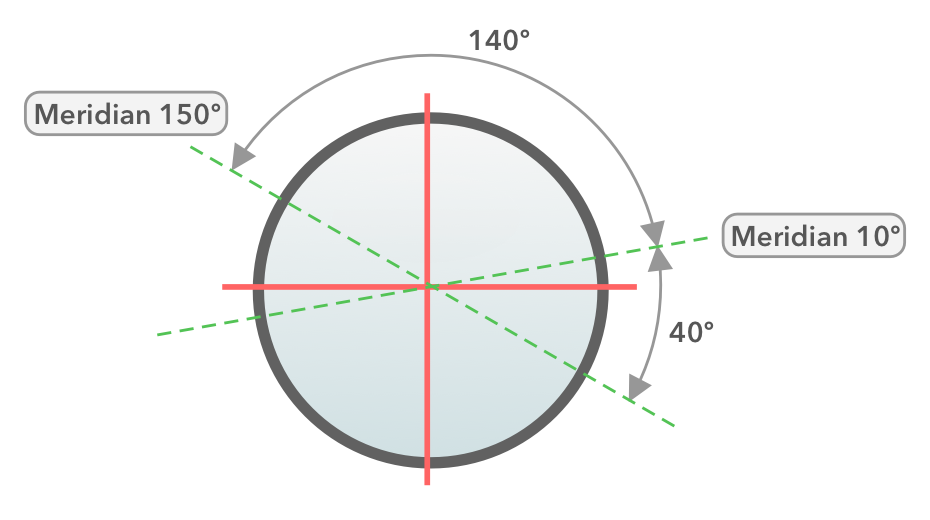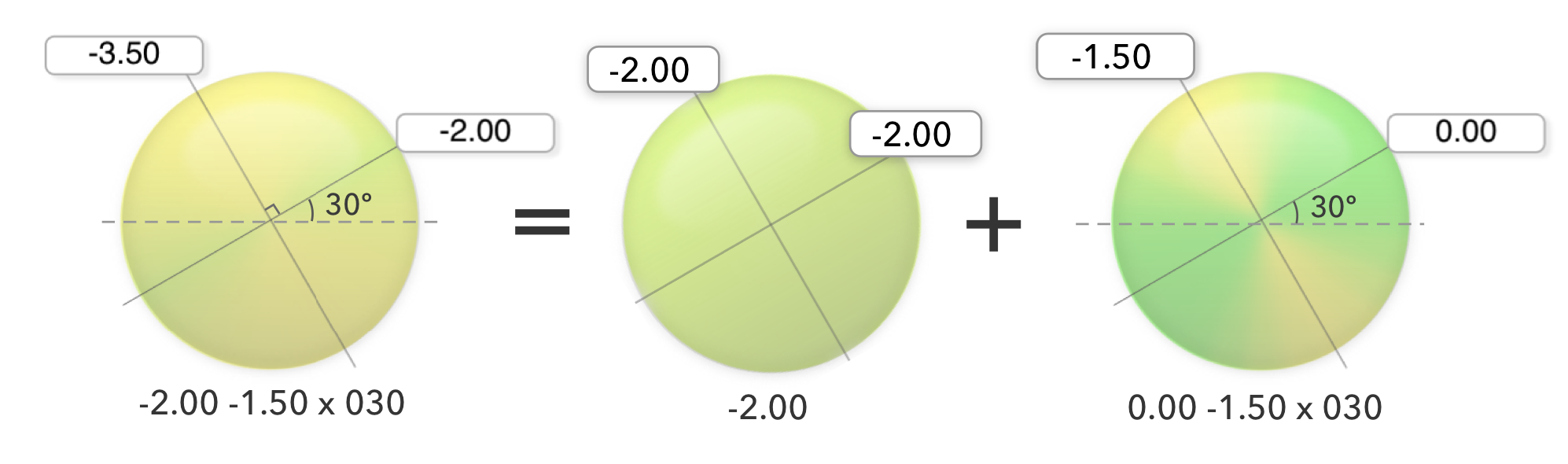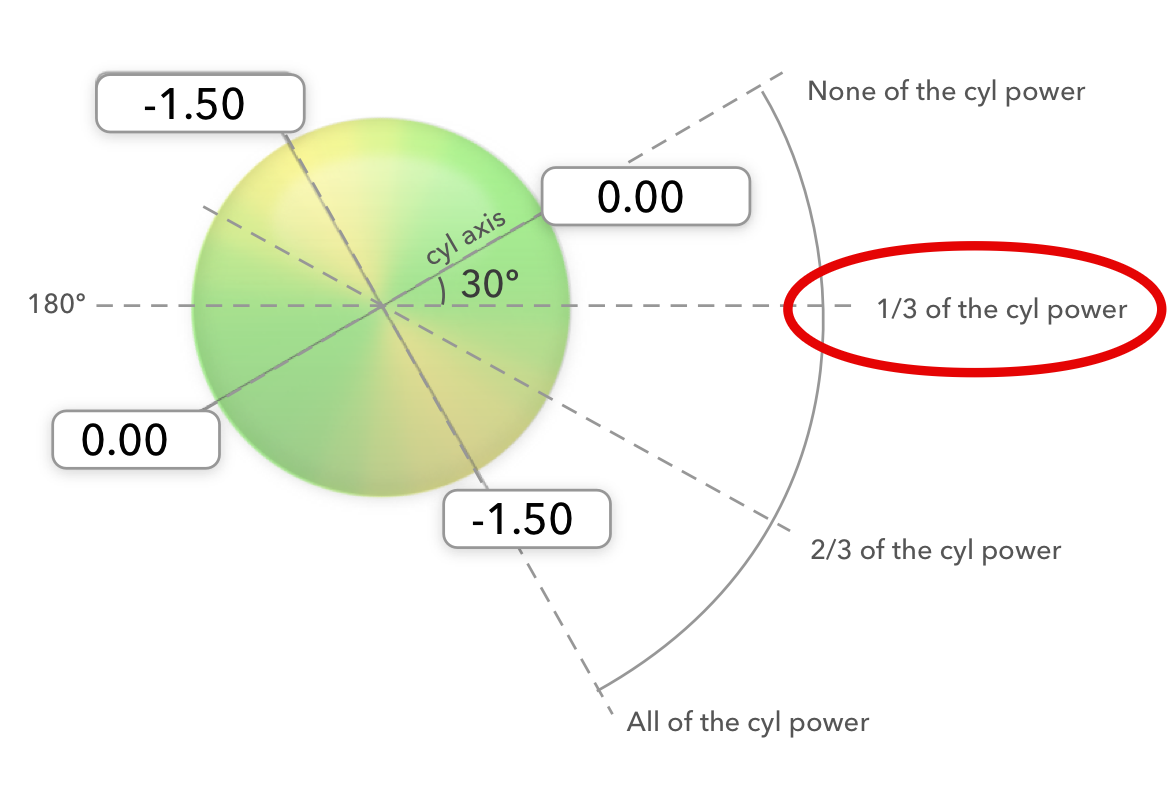# Discussing Meridian Power Calculations

I’ve added a new calculator to EyeDock that can determine the power in any meridian of a pair of spectacle lenses.It's located here, along with some accompanying text describing why such calculations might be useful (one of which includes insurance coverage for medically necessary contact lenses).

However, I wanted to expand a bit on how this calculation is done, and how the power in a meridian can be estimated if needed.

### Formula

First of all, getting the exact measurement of a meridian’s power is not difficult if you have the formula:

Dc´= Ds + Dc (sin² θ)

Where:

• Ds is the sphere component of the Rx
• Dc is the cylindrical power
• θ is the difference between the axis of the Rx and the meridian in question

Keep in mind that the theta (θ) value only needs to take into account the shortest distance between the cyl axis and the meridian of interest. For example, if our spectacle power is:

-2.00 -1.50 x 030

And we want to know the power at meridian 180º, subtracting the two will give us 180 - 30 = 150.

Of course, we all know meridian 180º is the same as meridian 0º, so the shortest distance between these two angles is 30 - 0 = 0º.

So, the rule of thumb is this: If you take the difference between the two angles and it’s bigger than 90º, subtract this number from 180º.

As another example, what is the difference between 150º and 10º?

150 - 10 = 140º

Since this result is greater than 90, subtract it from 180:

180 - 140 = 40º

So, the closest difference between meridian 10º and meridian 150º is 40º. To be honest, it’s probably easier to visualize this on an optical cross than to do it mathematically:### But I Can’t Remember Formulas!

So, what happens if you’re shipwrecked on a desert island and your survival depends on calculating a lens power in a specific meridian and you just can’t remember the above formula? Well, fortunately, this is actually pretty easy to estimate.

Let’s consider a spectacle Rx of:

-2.00 -1.50 x 030

And let’s suppose we want to know the power in the 180º meridian.

When thinking through optics calculations, I sometimes like to think of the Rx as split into a spherical component plus a cylindrical component, like this:For now, we can focus on the cylindrical component because this is the only where the power varies by the meridian (in other words, the spherical component isn’t very interesting because it has the same power in every meridian).

And what do we know about cylinder power? Well:

There is zero power at the cylinder’s axis, which is at 30º in this example.

100% of the cylinder power is located 90º away from the axis, which is at (30+90=) 120º.

For everything else, we can estimate the amount of cylinder as being proportional to how far you are in between these two meridians.

So, if we want to know the amount of cylinder in meridian 180, we just need to know how far this is from the cylinder axis. Meridian 180º is 30º away from axis 30º. Since the full cylinder is 90º away from our axis, 30/90 is ⅓ of the way there.⅓  of the total cyl power (-1.50) is -0.50.

Now that we know how much of our cylinder power is located at this meridian, we can add back in the spherical component of Rx:

-2.00 + -0.50 = -2.50 estimated total power in meridian 180º

That’s not exactly what we get when we use the formula, but it’s pretty darn close.